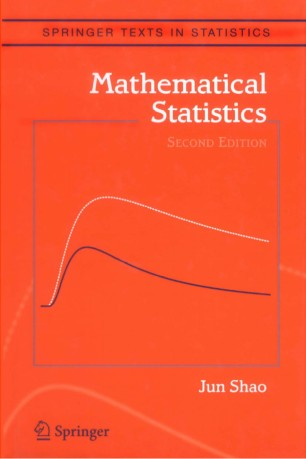# Mathematical Statistics

• Jun ShaoTextbook

Part of the Springer Texts in Statistics book series (STS)

1. Front Matter
Pages i-xvi
2. Pages 1-90
3. Pages 91-160
4. Pages 161-230
5. Pages 231-318
6. Pages 319-392
7. Pages 393-470
8. Pages 471-542
9. Back Matter
Pages 543-593

### Introduction

This graduate textbook covers topics in statistical theory essential for graduate students preparing for work on a Ph.D. degree in statistics. The first chapter provides a quick overview of concepts and results in measure-theoretic probability theory that are useful in statistics. The second chapter introduces some fundamental concepts in statistical decision theory and inference. Chapters 3-7 contain detailed studies on some important topics: unbiased estimation, parametric estimation, nonparametric estimation, hypothesis testing, and confidence sets. A large number of exercises in each chapter provide not only practice problems for students, but also many additional results.

In addition to improving the presentation, the new edition makes Chapter 1 a self-contained chapter for probability theory with emphasis in statistics. Added topics include useful moment inequalities, more discussions of moment generating and characteristic functions, conditional independence, Markov chains, martingales, Edgeworth and Cornish-Fisher expansions, and proofs to many key theorems such as the dominated convergence theorem, monotone convergence theorem, uniqueness theorem, continuity theorem, law of large numbers, and central limit theorem. A new section in Chapter 5 introduces semiparametric models, and a number of new exercises were added to each chapter.

### Keywords

Mathematical Statistics Statistical Theory likelihood Markov chain Mathematica mathematical statistics probability probability theory statistical theory statistics

• Jun Shao
• 1